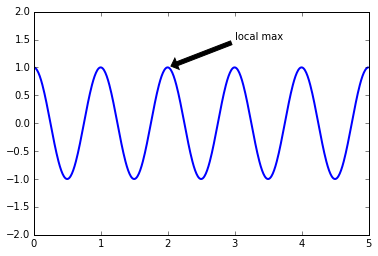# Pyplot 教程¶

## Matplotlib 简介¶

matplotlib 是一个 Python2D 图形包。

## 使用 Pyplot¶

In :
import numpy as np
import matplotlib.pyplot as plt


matplotlib.pyplot 包含一系列类似 MATLAB 中绘图函数的相关函数。每个 matplotlib.pyplot 中的函数对当前的图像进行一些修改，例如：产生新的图像，在图像中产生新的绘图区域，在绘图区域中画线，给绘图加上标记，等等…… matplotlib.pyplot 会自动记住当前的图像和绘图区域，因此这些函数会直接作用在当前的图像上。

## plt.show() 函数¶

plt.show() 默认是在新窗口打开一幅图像，并且提供了对图像进行操作的按钮。

• %matplotlib notebook
• %matplotlib inline

In :
%matplotlib inline


## plt.plot() 函数¶

### 例子¶

plt.plot() 函数可以用来绘图：

In :
plt.plot([1,2,3,4])
plt.ylabel('some numbers')

plt.show()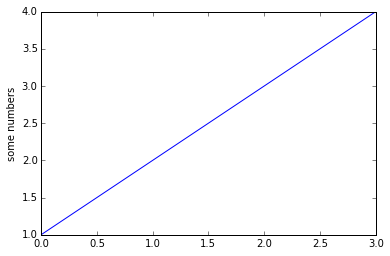### 基本用法¶

plot 函数基本的用法有以下四种：

• plt.plot(x,y)

• plt.plot(x,y, format_str)

• plt.plot(y)

• plt.plot(y, format_str)

In :
plt.plot([1,2,3,4], [1,4,9,16])

Out:
[<matplotlib.lines.Line2D at 0xa48a550>]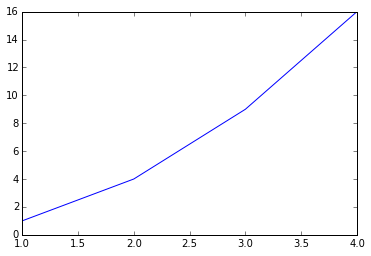### 字符参数¶

MATLAB 中类似，我们还可以用字符来指定绘图的格式：

‘b’ 蓝色，blue
‘g’ 绿色，green
‘r’ 红色，red
‘c’ 青色，cyan
‘m’ 品红，magenta
‘y’ 黄色，yellow
‘k’ 黑色，black
‘w’ 白色，white

'-' 实线 '--' 虚线
'-.' 虚点线 ':' 点线
'.' ',' 像素点
'o' 圆点 'v' 下三角点
'^' 上三角点 '<' 左三角点
'>' 右三角点 '1' 下三叉点
'2' 上三叉点 '3' 左三叉点
'4' 右三叉点 's' 正方点
'p' 五角点 '*' 星形点
'h' 六边形点1 'H' 六边形点2
'+' 加号点 'x' 乘号点
'D' 实心菱形点 'd' 瘦菱形点
'_' 横线点

In :
plt.plot([1,2,3,4], [1,4,9,16], 'ro')
plt.show()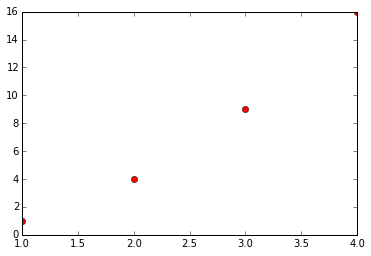### 显示范围¶

MATLAB 类似，这里可以使用 axis 函数指定坐标轴显示的范围：

plt.axis([xmin, xmax, ymin, ymax])
In :
plt.plot([1,2,3,4], [1,4,9,16], 'ro')
# 指定 x 轴显示区域为 0-6，y 轴为 0-20
plt.axis([0,6,0,20])
plt.show()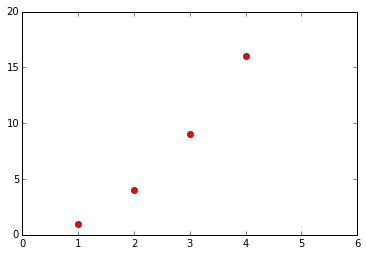### 传入 Numpy 数组¶

In :
import numpy as np
import matplotlib.pyplot as plt

# evenly sampled time at 200ms intervals
t = np.arange(0., 5., 0.2)

# red dashes, blue squares and green triangles
plt.plot(t, t, 'r--',
t, t**2, 'bs',
t, t**3, 'g^')

plt.show()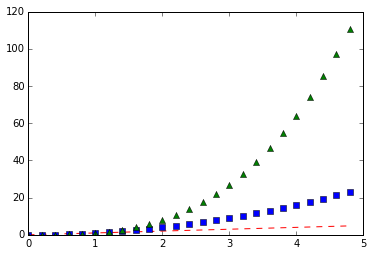### 线条属性¶

In :
x = np.linspace(-np.pi,np.pi)
y = np.sin(x)

plt.plot(x, y, linewidth=2.0, color='r')

plt.show()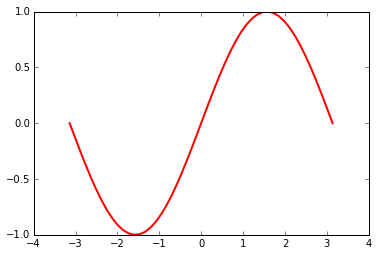### 使用 plt.plot() 的返回值来设置线条属性¶

plot 函数返回一个 Line2D 对象组成的列表，每个对象代表输入的一对组合，例如：

• line1, line2 为两个 Line2D 对象

line1, line2 = plt.plot(x1, y1, x2, y2)

• 返回 3 个 Line2D 对象组成的列表

lines = plt.plot(x1, y1, x2, y2, x3, y3)

In :
# 加逗号 line 中得到的是 line2D 对象，不加逗号得到的是只有一个 line2D 对象的列表
line, = plt.plot(x, y, 'r-')

# 将抗锯齿关闭
line.set_antialiased(False)

plt.show()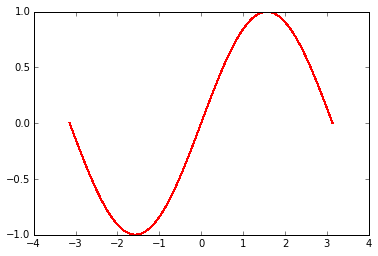### plt.setp() 修改线条性质¶

In :
lines = plt.plot(x, y)

# 使用键值对
plt.setp(lines, color='r', linewidth=2.0)

# 或者使用 MATLAB 风格的字符串对
plt.setp(lines, 'color', 'r', 'linewidth', 2.0)

plt.show()In :
plt.setp(lines)

  agg_filter: unknown
alpha: float (0.0 transparent through 1.0 opaque)
animated: [True | False]
antialiased or aa: [True | False]
axes: an :class:~matplotlib.axes.Axes instance
clip_box: a :class:matplotlib.transforms.Bbox instance
clip_on: [True | False]
clip_path: [ (:class:~matplotlib.path.Path,         :class:~matplotlib.transforms.Transform) |         :class:~matplotlib.patches.Patch | None ]
color or c: any matplotlib color
contains: a callable function
dash_capstyle: ['butt' | 'round' | 'projecting']
dash_joinstyle: ['miter' | 'round' | 'bevel']
dashes: sequence of on/off ink in points
drawstyle: ['default' | 'steps' | 'steps-pre' | 'steps-mid' |                   'steps-post']
figure: a :class:matplotlib.figure.Figure instance
fillstyle: ['full' | 'left' | 'right' | 'bottom' | 'top' | 'none']
gid: an id string
label: string or anything printable with '%s' conversion.
linestyle or ls: ['-' | '--' | '-.' | ':' | 'None' |                   ' ' | '']
linewidth or lw: float value in points
lod: [True | False]
marker: :mod:A valid marker style <matplotlib.markers>
markeredgecolor or mec: any matplotlib color
markeredgewidth or mew: float value in points
markerfacecolor or mfc: any matplotlib color
markerfacecoloralt or mfcalt: any matplotlib color
markersize or ms: float
markevery: [None | int | length-2 tuple of int | slice |         list/array of int | float | length-2 tuple of float]
path_effects: unknown
picker: float distance in points or callable pick function         fn(artist, event)
rasterized: [True | False | None]
sketch_params: unknown
snap: unknown
solid_capstyle: ['butt' | 'round' |  'projecting']
solid_joinstyle: ['miter' | 'round' | 'bevel']
transform: a :class:matplotlib.transforms.Transform instance
url: a url string
visible: [True | False]
xdata: 1D array
ydata: 1D array
zorder: any number


## 子图¶

figure() 函数会产生一个指定编号为 num 的图：

plt.figure(num)



plt.subplot(numrows, numcols, fignum)



numrows * numcols < 10 时，中间的逗号可以省略，因此 plt.subplot(211) 就相当于 plt.subplot(2,1,1)

In :
def f(t):
return np.exp(-t) * np.cos(2*np.pi*t)

t1 = np.arange(0.0, 5.0, 0.1)
t2 = np.arange(0.0, 5.0, 0.02)

plt.figure(1)
plt.subplot(211)
plt.plot(t1, f(t1), 'bo', t2, f(t2), 'k')

plt.subplot(212)
plt.plot(t2, np.cos(2*np.pi*t2), 'r--')
plt.show()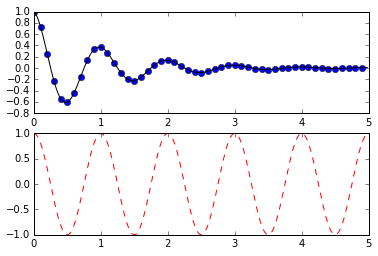## 图形上加上文字¶

plt.hist() 可以用来画直方图。

In :
mu, sigma = 100, 15
x = mu + sigma * np.random.randn(10000)

# the histogram of the data
n, bins, patches = plt.hist(x, 50, normed=1, facecolor='g', alpha=0.75)

plt.xlabel('Smarts')
plt.ylabel('Probability')
plt.title('Histogram of IQ')
plt.text(60, .025, r'$\mu=100,\ \sigma=15$')
plt.axis([40, 160, 0, 0.03])
plt.grid(True)
plt.show()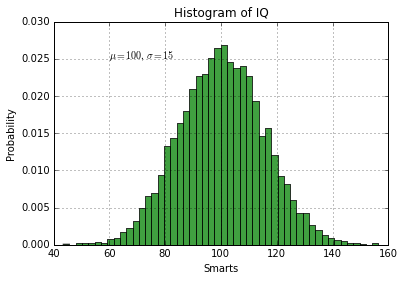• xlabel ：x 轴标注

• ylabel ：y 轴标注

• title ：图形标题

• text ：在指定位置放入文字

• xy ：注释位置
• xytext ：注释文字位置
In :
ax = plt.subplot(111)

t = np.arange(0.0, 5.0, 0.01)
s = np.cos(2*np.pi*t)
line, = plt.plot(t, s, lw=2)

plt.annotate('local max', xy=(2, 1), xytext=(3, 1.5),
arrowprops=dict(facecolor='black', shrink=0.05),
)

plt.ylim(-2,2)
plt.show()